More results...

Generic selectors
Exact matches only
Search in title
Search in content
Post Type Selectors

# Gold Leaf Electroscope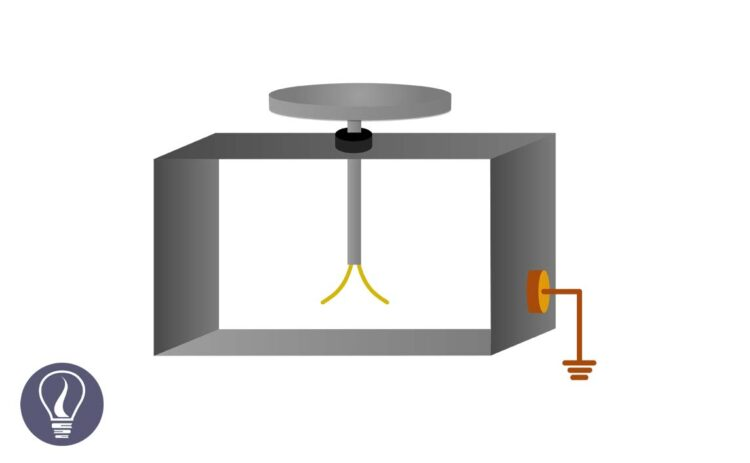B.Sc (Hons, USJ) (Polymer Science and Technology, Chemistry, Physics)
Categories
Last Updated On : September 20, 2023
Published Date : September 2, 2023

Gold leaf electroscope is used to detect the electric charge of an object.

In the gold leaf electroscope, there is a metal plate and it is attached to a metal rod. Two light gold sheets are attached to the other end of the rod. This apparatus is placed in a glass case, as the metal plate is located outside and the metal rod and the gold leaf are placed inside the glass case. The glass case is sealed with an insulating material.

## How does the gold leaf electroscope work?

Metal plates, metal rods, and the two gold leaves are conductive materials. Therefore, electrons can freely move through them. A gold leaf electroscope should be neutralized before using it. The electroscope is grounded to neutralize. This can be done by simply touching the metal plate of the electroscope.

If the electroscope is already negatively charged, the excess electrons will be grounded. If the electroscope is already positively charged, electrons will be added to the electroscope to neutralize the positive charge.

When the gold leaf electroscope has a charge, two gold leaves show a divergence. If the charge is high, the angle between the two gold leaves is high. When the electroscope is neutralized, the angle between the gold leaves is zero.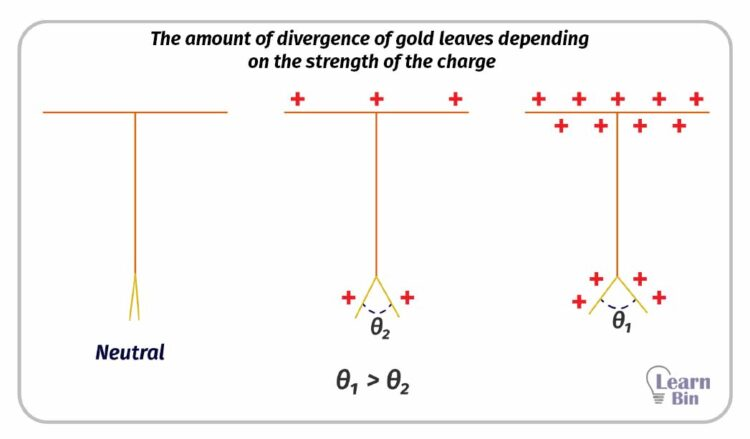Figure 03: The amount of divergence of gold leaves depending on the strength of the charge

When a charged object is brought closer to the neutralized gold leaf electroscope, we can observe a divergence between the two gold leaves. If the electroscope shows a small angle between two gold leaves even after the object is touched and then taken away, the object is a conductor. This observation can be explained as follows.

### a. What happens when a positively charged object is brought closer to the neutral gold leaf electroscope

When a positively charged object is brought closer to the metal plate of the gold leaf, the negative charges (electrons) in the electroscope move toward the metal plate. Therefore, the two gold leaves are charged positively. Since the like charges repel each other, the two gold leaves repel each other. Therefore, it shows an angle between the two gold leaves.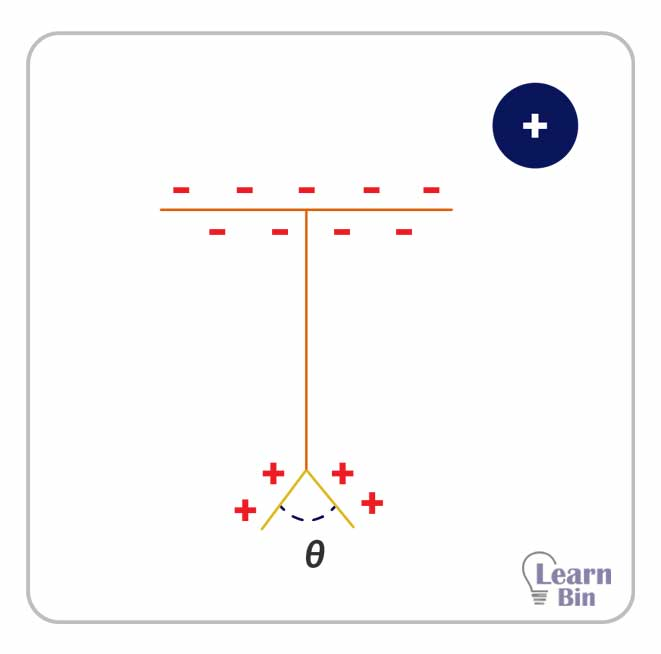Figure 04: What happens when a positively charged object is brought closer to the neutral gold leaf electroscope

### b. What happens when a negatively charged object is brought closer to the neutral gold leaf electroscope

When a negatively charged object is brought closer to the metal plate of the gold leaf, the negative charges (electrons) in the electroscope move toward the gold leaves. Therefore, the two gold leaves are charged negatively. Since the like charges repel each other, the two gold leaves repel each other. Therefore, it shows an angle between the two gold leaves.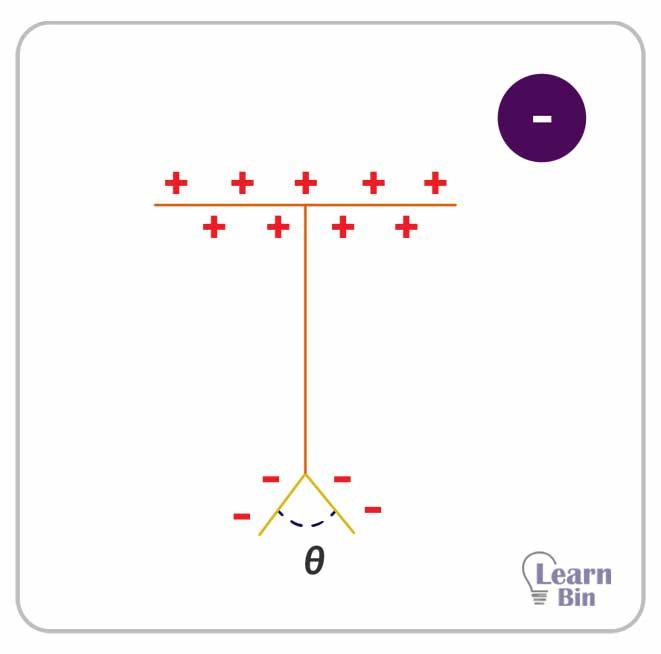Figure 05: What happens when a negatively charged object is brought closer to the neutral gold leaf electroscope

### c. What happens when the object is touching the metal plate

If the object is a conductor, according to the charge of the object, electrons will move from the electroscope to the object or from the object to the electroscope.

## How to charge the gold leaf electroscope

The gold leaf electroscope can be charged positively or negatively using a charged object. If we use a positively charged object, the electroscope can be charged negatively. If we use a positively charged object, the electroscope can be charged negatively.

### a. Charging the electroscope positively

To charge the electroscope positively, we have to use a negatively charged object. An ebonite rod can be charged positively by rubbing it with a wool cloth.

• First, the negatively charged ebonite rod is brought closer to the metal plate. Since the ebonite rod is negatively charged, the negative charges of the electroscope move toward the gold leaves. Therefore, the negative charge density is increased around the two gold leaves. So, the two gold leaves are repulsed by each other.
• While keeping the ebonite rod near, the metal plate is grounded. When the metal plate is grounded, the negative charges around the two gold leaves are grounded. Therefore, the angle between the two gold leaves is decreased.
• While keeping the ebonite rod, the grounded connection is disconnected. After disconnecting, the ebonite rod is taken away. Now, the electroscope has charged positively. After the ebonite rod has been removed, the charges in the electroscope spread throughout the electroscope evenly. Two gold leaves show an angle between them.

### b. Charging the electroscope negatively

To charge the electroscope negatively, we have to use a positively charged object. A glass rod can be charged positively by rubbing it with a silk cloth.

• First, the negatively charged rod is brought closer to the metal plate. Since the glass rod is positively charged, the negative charges of the electroscope move towards the metal plate. Therefore, the positive charge density is increased around the two gold leaves. So, the two gold leaves are repulsed by each other.
• While keeping the glass rod near, the metal plate is grounded. When the metal plate is grounded, the excess negative charges from the ground move toward the two gold leaves. Therefore, the angle between the two gold leaves is decreased.
• While keeping the glass rod, the grounded connection is disconnected. After disconnecting, the glass rod is taken away. Now, the electroscope has charged negatively. After the glass rod has been removed, the charges in the electroscope spread throughout the electroscope evenly. Two gold leaves show an angle between them.

## How to find the charge of an object using a gold leaf electroscope

When a charged object is brought closer to a charged electroscope, if the angle between the two gold leaves is increased, the object has the same charge as the electroscope. If the angle decreases the object has the opposite charge as the electroscope. This observation can be described as follows.

When a positively charged object is brought closer to a positively charged electroscope, the negative charges around the gold leaves move toward the metal plate. Therefore, the positive charge density of the gold leaves is further increased. So, the angle between the two gold leaves is further increased.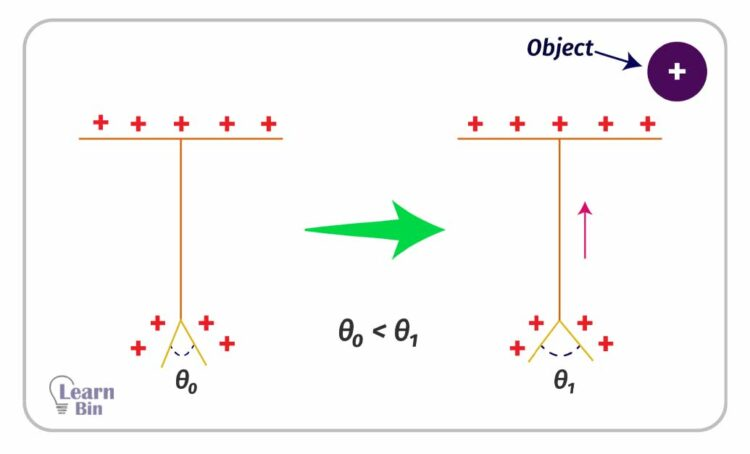Figure 10: How to find the charge of an object using a gold leaf electroscope | positive object - positive electroscope.

When a negatively charged object is brought closer to a positively charged electroscope, the negative charges around the metal plate move toward the gold leaves. Therefore, the positive charge density of the gold leaves is decreased. So, the angle between the two gold leaves is decreased.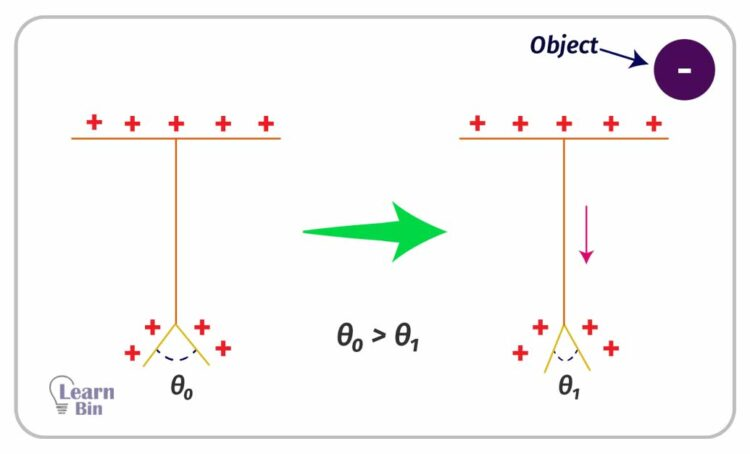Figure 11: How to find the charge of an object using a gold leaf electroscope | positive object - negative electroscope.

When a negatively charged object is brought closer to a negatively charged electroscope, the negative charges around the metal plate move toward the gold leaves. Therefore, the negative charge density of the gold leaves is further increased. So, the angle between the two gold leaves is further increased.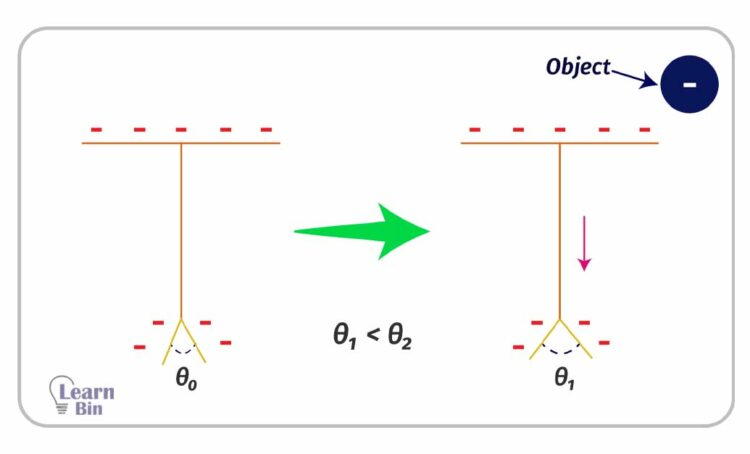Figure 12: How to find the charge of an object using a gold leaf electroscope | negative object - negative electroscope.

When a positively charged object is brought closer to a negatively charged electroscope, the negative charges around the gold leaves move toward the metal plate. Therefore, the negative charge density of the gold leaves is decreased. So, the angle between the two gold leaves is decreased.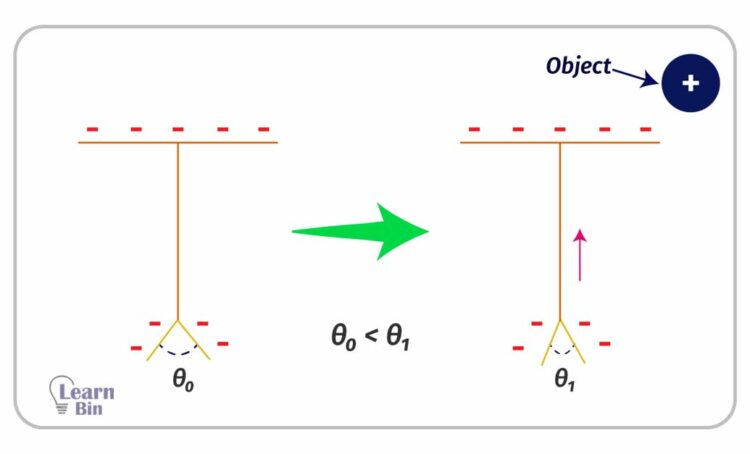Figure 13: How to find the charge of an object using a gold leaf electroscope | positive object - negative electroscope.

## Measuring potential difference using gold leaf electroscope

Gold leaf electroscope can be used as a volt meter to measure static voltage. When a capacitor is connected to a neutral electroscope, two gold leaves show an angle. This angle is proportional to the voltage of the capacitor.

When this electroscope is connected to a capacitor with a known voltage,

Where θ is the angle between two gold leaves and V is the potential difference.## References and Attributes

### Figures:

The cover image was designed using an image by Malyszkz, licensed under CC BY-SA 3.0, via Wikimedia Commons